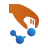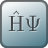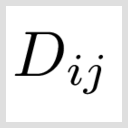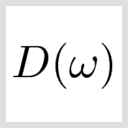# Dynamical Matrix study object: Phonons in bulk silicon¶

Version: P-2019.03

## Introduction¶

In this tutorial you will learn how to obtain the dynamical matrix using the DynamicalMatrix study object, and compute the phonon band structure and density of states. In particular, you will learn how to set up the calculations required for the dynamical matrix for force-field engines, taking bulk silicon as an example.

• You will use the ATK-ForceField engine for very fast calculations of the silicon dynamical matrix, and visualize the phonon band structure and phonon DOS.

## Phonons¶

The dynamical matrix is a central quantity of lattice dynamics: For each atom in the system, it gives the force response to displacements of other atoms away from equilibrium, i.e. the first derivative of forces wrt. atom displacements. Phonon frequencies (quantized lattice vibrations) are calculated from the eigenvalues of the dynamical matrix. In QuantumATK, the dynamical matrix is calculated using a finite-difference scheme, where each symmetrically unique atom is displaced along all cartesian directions, also called frozen phonon calculations.

For crystals with small unit cells, a periodically repeated supercell of the unit cell is often needed to accurately account for long-range interactions in the dynamical matrix, and is therefore needed to obtain reliable phonon band structure and DOS. Importantly, the DynamicalMatrix study object can handle this automatically: By default, the number of repetitions along each lattice vector is set such that each atom is at least 4 covalent bond radii away from its translated copies. This usually includes atoms that are up to 4 bonds away, which is suitable for most systems. You can see more details in notes of the manual entry for DynamicalMatrix.

## ATK-ForceField¶

The ATK-ForceField calculator engine includes a large set of classical potentials which are well suited to study vibrational properties of materials, such as the phonon band structure and phonon density of states of silicon.

Go to theBuilder and import the Silicon (alpha) structure from the built-in database. Then send the structure to theScript Generator and add the following script blocks:

1.ForceFieldCalculator
2.OptimizeGeometry
3.DynamicalMatrix
4.PhononBandstructure
5.PhononDensityOfStatesTip

### Simulation settings¶

In theForceFieldCalculator settings, select an appropriate potential available for silicon, such as the Tersoff [GdGPB91] or the Stillinger–Weber potentials [SW85]. Each potential is provided with a literature reference, which you should in general check to determine if the potential is well suited for your system and for the property you are interested in.In theOptimizeGeometry settings, untick the option Fix Lattice Vectors to relax also the lattice constants, and decrease the force and stress tolerances to 0.001 eV/Å and 0.0001 eV/Å3, respectively.In theDynamicalMatrix settings, keep the default settings. This will automatically create an appropriately sized supercell and will enable acoustic sum rule.In thePhononBandstructure settings, increase the points to 100 and setup the Brillouin zone route as G, K, X, G, L, X, W, L to compare to reference [GdGPB91].

Change the default output filename to Silicon.hdf5, and send the script to theJob Manager and run it. The calculation will take just a few seconds on a normal desktop machine.

### Results¶

See your LabFloor and visualize the phonon band structure and phonon density of states using the PhononBandstructure Analyzer and DOS Analyzer plug-ins. The phonon band structure of silicon calculated with the Tersoff_Si_2005 potential is reported in the figure below.You can also see the size of the automatically chosen supercell in the log file as the following below:

+----------------------------------------------------------+
| Bulk: Cartesian (Angstrom) / fractional                  |
+----------------------------------------------------------+
2
Bulk
Si    0.000000e+00  0.000000e+00  0.000000e+00    0.00000  0.00000  0.00000
Si    1.357650e+00  1.357650e+00  1.357650e+00    0.25000  0.25000  0.25000
+------------------------------------------------------------------------------+
| Automatically detected repetitions = [7 7 7]                                 |
+------------------------------------------------------------------------------+
+------------------------------------------------------------------------------+
| Dynamical Matrix Study                                                       |
+------------------------------------------------------------------------------+
| 2 task(s) will be executed.                                                  |
|                                                                              |
| * Bundled force calculation                                                  |
|   Displacements: 0-10                                                        |
| * Generate dynamical matrix from displaced forces                            |
+------------------------------------------------------------------------------+
+------------------------------------------------------------------------------+
| Executing task 1 / 2:                                                        |
|   Bundled force calculation                                                  |
|   Displacements: 0-10                                                        |
|   Log to: stdout                                                             |
+------------------------------------------------------------------------------+
+------------------------------------------------------------------------------+
| Executing task 2 / 2:                                                        |
|   Generate dynamical matrix from displaced forces                            |
|   Log to: stdout                                                             |
+------------------------------------------------------------------------------+